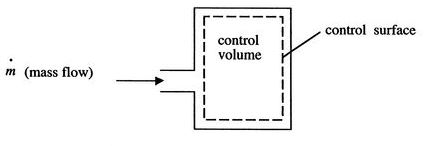First law of thermodynamics

Energy can be changed from one form to another, but it cannot be created or destroyed. The total amount of energy and matter in the Universe remains constant, merely changing from one form to another. The First Law of Thermodynamics (Conservation) states that energy is always conserved, it cannot be created or destroyed. In essence, energy can be converted from one form into another.

The First Law of Thermodynamics states that energy can be converted from one form to another with the interaction of heat, work and internal energy, but it cannot be created nor destroyed, under any circumstances. Mathematically, this is represented as

ΔU= Q - W
where ,
• ΔU is the total change in internal energy of a system,
• Q is the heat exchanged between a system and its surroundings, and
• w is the work done by or on the system.

•